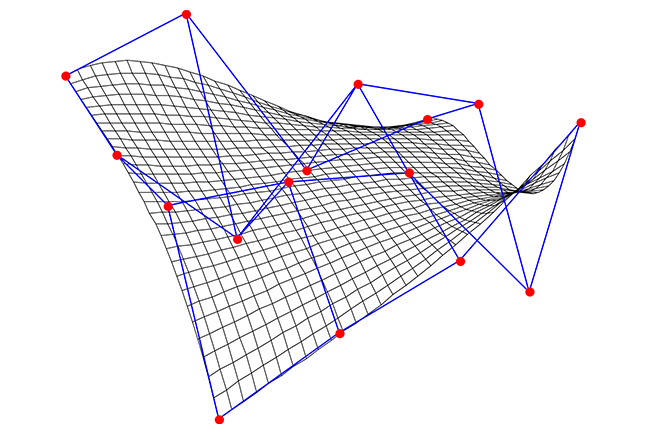## Want to keep learning?

This content is taken from the UNSW Sydney's online course, Maths for Humans: Linear and Quadratic Relations. Join the course to learn more.
4.10

## UNSW SydneyBézier surface example

# Parametric forms for lines and vectors

In many situations, it is useful to have an alternative way of describing a curve besides having an equation for it in the $$\normalsize{x-y}$$ plane. A parametric form for a line occurs when we consider a particle moving along it in a way that depends on a parameter $$\normalsize{t}$$, which might be thought of as time. Thus both $$\normalsize{x}$$ and $$\normalsize{y}$$ become functions of $$\normalsize{t}$$. The simplest parameterisation are linear ones.

In this step we

• look at parameterisations of the simplest curves: lines

• show how to go from parametric equations to Cartesian equations

• introduce vectors.

## Parameterisations of a line

A simple example of a parameterisation is a linear parameterisation:

$\Large{p(t)=[t+1, 2t-3]}.$

As $$\normalsize{t}$$ varies, the point $$\normalsize{p(t)}$$ moves along a line. When $$\normalsize{t=0}$$, $$\normalsize{p(0)=[1,-3]}$$, when $$\normalsize{t=1}$$, $$\normalsize{p(1)=[2,-1]}$$, and when $$\normalsize{t=2}$$, $$\normalsize{p(2)=[3,1]}$$. Clearly also fractional values of $$\normalsize{t}$$ are allowed.How do we go from a parametric form for a line to one of the more usual Cartesian forms involving $$\normalsize{x}$$ and $$\normalsize{y}$$? We eliminate the parameter $$\normalsize{t}$$. For example for the parametric equation $$\normalsize{p(t)=[t+1, 2t-3]}$$ we have

$\Large{x=t+1}$ $\Large{y=2t-3}$

so that $$\normalsize{y=2(x-1)-3}$$ or $$\normalsize{y=2x-5}$$. Please check that this is the same line – with a different equation.Q1 (E): Find a Cartesian equation for the parametric line $${\normalsize p(s) = [2s+1, 4s-3]}$$.

## How to go from a Cartesian equation to a parametric form?

On the other hand, how can we go from a Cartesian equation of a line, say $$\normalsize{y=3x+2}$$ to a parametric form? It is important to realise that although the Cartesian form is more or less unique, this is not at all the case for a parametric form.

One way is to introduce a parameter in a simple way, say by setting $$\normalsize{x=t}$$. Then the original equation gives $$\normalsize{y=3x+2=3t+2}$$. Putting these together gives the parameterisation $$\normalsize{p(t)=[t,3t+2]}$$.

Q2 (E): Find another parameterisation of $$\normalsize y=3x+2$$ by setting $$\normalsize x=3t$$.

## Using vectors to parameterise lines

The rest of this step is about vectors, for those who are familiar with them. A vector is a directed line segment, or geometrically a difference between two points in the plane. We will denote vectors by round brackets, such as $$\normalsize{v=(1,4)}$$. This represents a relative displacement of $$\normalsize{1}$$ to the right in the $$\normalsize x$$-direction and $$\normalsize{4}$$ up in the $$\normalsize y$$-direction. This plays a similar role to the notion of slope, which is the ratio of the relative displacement of the $$\normalsize x$$ and $$\normalsize y$$-directions or “rise over run”. Using vectors allows us to be more precise about the notion of direction of a line.

We agree that vectors are not fixed in place, as they are only determined in a relative sense.

Suppose that we consider the line through the point $$\normalsize{[3,2]}$$ which goes in the direction $$\normalsize{v=(1,4)}$$. This line can be expressed as

$\Large{[3,2]+t(1,4)=[3+t,2+4t]}$

which is now in parametric form with parameter $$\normalsize{t}$$.

What is the Cartesian equation of this line? We may eliminate $$\normalsize{t}$$ from the equations $$\normalsize{x=3+t}$$, $$\normalsize{y=2+4t}$$ to get $$\normalsize{y=2+4(x-3)=4x-10}$$ so the equation is $$\normalsize{y=4x-10}$$.Now imagine that we would like to traverse the same line, only faster. To move through the same line at double the speed, we replace $$\normalsize{t}$$ with $$\normalsize{2t}$$:

$\Large{[3,2]+2t(1,4)=[3,2]+t(2,8)}.$

Notice that the direction vector has doubled, but the Cartesian equation of this line remains unchanged. We can also move backwards along the line by relpacing $$\normalsize{t}$$ with $$\normalsize{-t}$$:

$\Large{[3,2]-t(1,4)=[3,2]+t(-1,-4)}.$

Now the direction vector has been negated, but again the Cartesian equation remains unchanged.

So we see that the parameterisation of a curve describes more than the Cartesian equation, a parameterisation describes how we traverse the curve: fast or slow, forwards or backwards.

A1. This is also $$\normalsize{y=2x-5}$$.
A2. Another parameterisation is $$p(t) = [3t, 9t+2]$$.